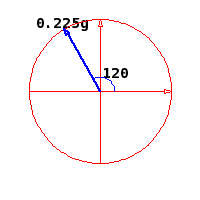# Balance the force to find the angle

## Homework Statement

A mass, m = 175 gram is placed on a hanger which has a mass of 50 g, giving a total mass of 225 g. The hanger is then hung at an angle of 120° over a pulley attached to the force table by using a string of negligible mass. The pulley over which the string rests has very low frictional force.In order to balance the force F another mass needs to be hung from the force table. What is the total mass (mass plus hanger) needed to balance the force F? At what angle should the mass be hung? Give your angle from the positive x-axis.

Tx=Tcos(theta)
Ty=Tsin(theta)
A=SQRT(X^2+Y^2)
T= mg

## The Attempt at a Solution

T = 2.2 N from .225*9.8
Tx=2.2cos120=-1.10N
Ty=2.2cos120=1.91N

Mass to balance = 225g
Angle = -30degrees?

the way I was told to find the angle was to draw the negative of the angle, so I drew the vector on the opposite side of the 2nd quadrant onto the 4th quadrant. Then find the angle according to the +x-axis. I drew the angle and for me I got -30 degrees since I assumed that since the 1st quadrant is 90degrees, then the remaining degree on the 2nd quadrant is obviously 30 and the opposite of it into the 4th quadrant would be -30?

Any hints/help would be appreciated. Thanks

edit :Wow - nevermind... I figured this out lol. Ignoring this problem and coming back to it an hour later works wonders :P

Last edited:

## Answers and Replies

Hey....how'd you figure it out? I'm stuck at the same place and with your previous answers lol. Any help would be appreciated